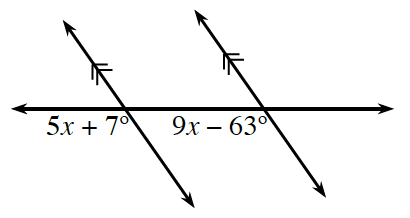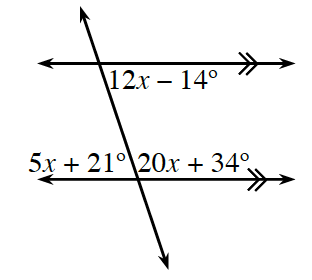### Home > INT2 > Chapter 2 > Lesson 2.1.2 > Problem2-22

2-22.

Use your knowledge of angle pair relationships to write an equation and solve for $x$ in the diagrams below. Then calculate the measures of the labeled angles. Justify your solutions by naming the theorem.

1.corresponding angles

$5x + 7 = 9x - 63$

1.Multiple relationships can be used to solve for $x$.